Applied and Computational Mathematics
Volume 5, Issue 3, June 2016, Pages: 160-164

The Numerical Solution of the TVD Runge-Kutta and WENO Scheme to the FPK Equations to Nonlinear System of One-Dimension

Wang Wenjie1, Feng Jianhu1, Xu Wei2

1School of Science of Chang’an University, Xi’an, China

2School of Science of Northwestern Polytechnic University, Xi’an, China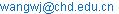(Wang Wenjie)

Wang Wenjie, Feng Jianhu, Xu Wei. The Numerical Solution of the TVD Runge-Kutta and WENO Scheme to the FPK Equations to Nonlinear System of One-Dimension. Applied and Computational Mathematics. Vol. 5, No. 3, 2016, pp. 160-164. doi: 10.11648/j.acm.20160503.20

Received: June 19, 2016; Accepted: June 27, 2016; Published: July 23, 2016

Abstract: Firstly, it was studied to the Fokker-Planck-Kolmogorov (FPK) equations for nonlinear stochastic dynamic system. Secondly, it was discussed to the third-order TVD Runge-Kutta difference scheme totime for differitial equations and the fifth-order WENO scheme for differitial operators. And combined he third-order TVD Runge-Kutta difference scheme with the fifth-order WENO scheme, obtained the numerical solution for FPK equations using the TVD Runge-Kutta WENO scheme. Finally, the numerical solution was compared with the analytic solution for FPK equations. The numerical method is shown to give accurate results and overcomes the difficulties of other methods, such as: the big value of probability density function at tail etc.

Keywords: Nonlinear System, FPK Equations, The Finite Difference Method, The TVD Runge-Kutta Scheme, The ENO Scheme, The WENO Scheme

1. Introduction

The FPK equation was first proposed by Fokker and Planck in 20 Century, and was applied to the study of quantum physics. At the beginning of 1930s it is generalizated and abstracted by Kolmogorov. Soon, it is applied to the study of general dynamic systems by Andronov et al. In the 50's Stratonovich applied it to the study of electronic engineering problems. At the end of the 50's Chuang and Kazda applied it to the study of nonlinear control systems. In the early 60's, it is applied to the study of nonlinear random vibration problems by Ariaratnam, Lyon, Smith, Caughey and Dienes, Crandall and so on.

The response and reliability of the system can be qualitatively analyzed by using the transition probability density of the system. However, at present, only some special nonlinear stochastic dynamical systems can get the exact solution of the FPK equation [1-3]. Based on this, many scholars dedicated to the study on the numerical solution of FPK equation, which represents the main finite element method and finite difference method, the path integral method, equivalent linearization method, Gaussian closure method, perturbation method, the gram Charlier expansion method, equivalent nonlinear system method, stochastic averaging method. However, these methods have their shortcoming: the Calculation quantity of the finite element method is usually very large, and the tail probability density is not accurate; equivalent linearization method and Gauss's method is not applicable to strongly nonlinear [4-6] systems or systems with random parametric excitation, for the steady-state probability density of the system response is often non Gauss type; perturbation method is only applicable to weakly nonlinear systems; the Gram-Charlier expansion method may lead to the case that the probability density is negative; equivalent nonlinear system method requires that the properties of the two nonlinear systems are very close; the stochastic averaging method is only applicable to the case of weak damping and weak excitation.

And weighted essentially non oscillatory (WENO) method is in recent years popular a kind of high resolution numerical method, for solving convection dominated convection diffusion equation, especially for those of hyperbolic conservation laws. WENO method is structured by the weighted idea based on the ENO method to solve problem of the shock, sparse and contact discontinuities and other complex structure of the fluid problem. The performance of WENO method is more stable and the convergence is better. It can guarantee that the accuracy of the smooth region of the solution is higher, and the discontinuous in the solution maintain the steep discontinuity and the nature of the oscillation. WENO scheme is initially proposed and constructed a third order finite volume WENO scheme in 1994 by Liu, Osher and Chan, which uses the convex combination of all of the candidate templates and is different in the eno format single template selection, and systematically discusses the WENO method of construction process and theoretical analysis. Then, the framework is given in which can construct arbitrary precision finite difference WENO scheme in the multidimensional space, in 1996, Jiang and Shu, and in which a fifth order accuracy WENO scheme was designed, and the smooth factor and the basic structure of nonlinear weight. So far, five order WENO scheme is the most widely used, which is the standard formats for solving WENO reconstruction process of the hyperbolic conservation laws equation.

In this paper, a new TVD Runge Kutta WENO type difference method is proposed for based the above problems, combined with the third order TVD Runge Kutta method, better than other method for solving FPK equation, and can obtained more accurately the probability density function.

2. The FPK Equation of the Nonlinear Dynamic System with Random Excitation

For nonlinear stochastic dynamical systems, the corresponding FPK equations have the following form: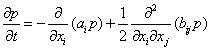(1)

FPK equation (1) is a variable coefficient parabolic partial differential equation, which describes the evolution or the flow of the transition probability density of the diffusion process, in which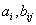is the drift and diffusion coefficient Correspondingly.

Whennot contains time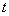obviously, the FPK equation (1) can be transferred to: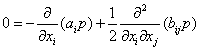(2)

FPK equation (2) is a partial differential equation with variable coefficients, which is often referred to as a simplified or stationary FPK equation, whose solution will be stationary probability density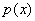. To uniquely determine the solution of the FPK equation (1), the initial and boundary conditions are required.

In this paper, we use the following initial conditions: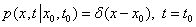(3)

which expressed that the system is in the initial state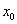with probability 1 at the moment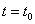.

Infinite boundary condition: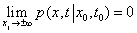(4)

Or, it must at least to close to zero by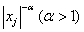.

In the random vibration theory, the FPK equation (1) and (2) are commonly used to predict the response of nonlinear stochastic dynamic systems. Therefore, the accuracy of the solution of the FPK equation plays an important role in the reliability analysis.

3. The Finite Difference Method for FPK Equation

3.1. Runge-Kutta TVD Scheme

Let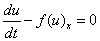is a hyperbolic partial differential equation in a general form, and the semi discrete scheme about space is: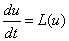(5)

In which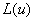is the approximation of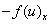. For the semi discrete form equation (5), the standard Runge-Kutta time discretization achieves the stability of the format basing on the linear stability conditions to, here the CFL can take a larger value. But for the nonlinear equations, CFL must be very small in order to ensure the stability of the scheme. Due to coupling the high order spatial discretization and low order Runge Kutta time discrete, CFL must greatly lower than the requirements of the linear stability, in order to guarantee the discrete scheme with high order accuracy and non oscillatory. In this paper, we use the three order Runge-Kutta time discretization scheme with TVD properties :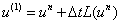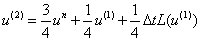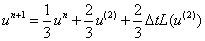Where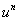is the conservation of the time layer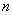, andis the difference operator after space discretization.

3.2. The Five-Order WENO Scheme

Let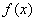to be a function,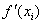can use the five - order WENO scheme: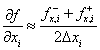(6)

In which,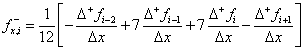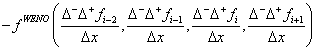,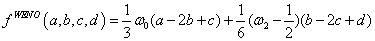,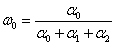,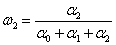,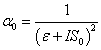,,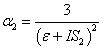,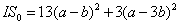,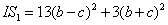,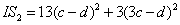,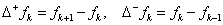Where,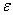is a small amount.

Samely,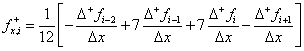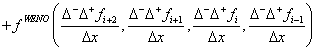.

And for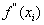, the four order central difference scheme [8-10] is used: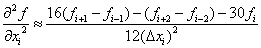(7)

In which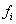represents the value of the functionat the point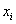,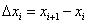.

3.3. The Runge-Kutta Weno TVD Type Difference Scheme for the FPK Equation

For one dimensional FPK equation (1), the Runge-kutta Weno TVD type difference scheme of one-dimensional FPK equation can be obtained by combining the three order Runge-kutta TVD form and the five order WENO scheme of the differential equation.

The Runge-kutta Weno TVD type difference scheme for one dimensional problem:(8)

Where,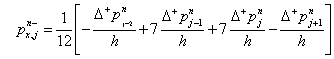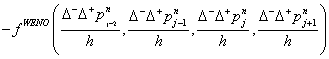,,,,,,,,,,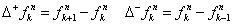In which,is a small amount.

And,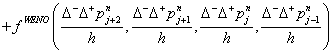.

where,represents the corresponding value of the function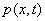at the point,is the size of the space step and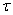is the siza of the time step.

4. Numerical Examples

Example 1. A single degree of freedom nonlinear system with random external excitation is considered: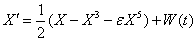(9)

Where,is a constant representating the intensity of nonlinear systems,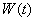is a zero mean white Gauss noise, its correlation coefficient is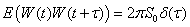,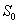is the spectral density of, and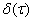is the Dirac function.

When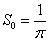, the FPK equation of the steady-state probability densityof the response of corresponding to the system (9) is: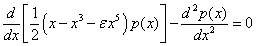(10)

The solution is:(11)

In which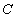is the normalized constant. The exact solution is compared with the numerical solution of the method in this paper, as shown in figure 1.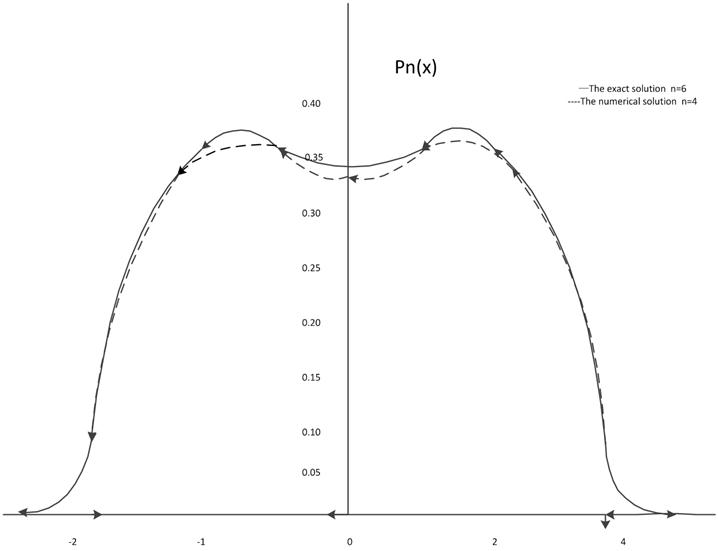Fig. 1. The exact solution and the numerical solution.

Example 2. The nonlinear oscillator under the combined action of Gauss white noise and external excitation is considered: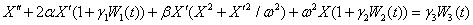(12)

where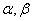is constant,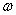is an normal number,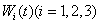is the Gauss white noise with zero mean, and independent of each other, which satisfy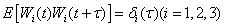,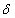is the Dirac function,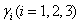represents the noise intensity.

When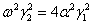, the exact steady state solution is existed for the corresponding FPK equation of the system (2). That is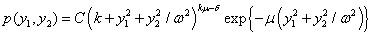,

in which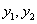indicates the displacement and speed of response, andis the normalized constant,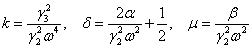. The exact solution of the edge probability density of the displacement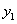and velocity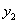is compared with the numerical solution of the method in this paper, as shown in figure 2.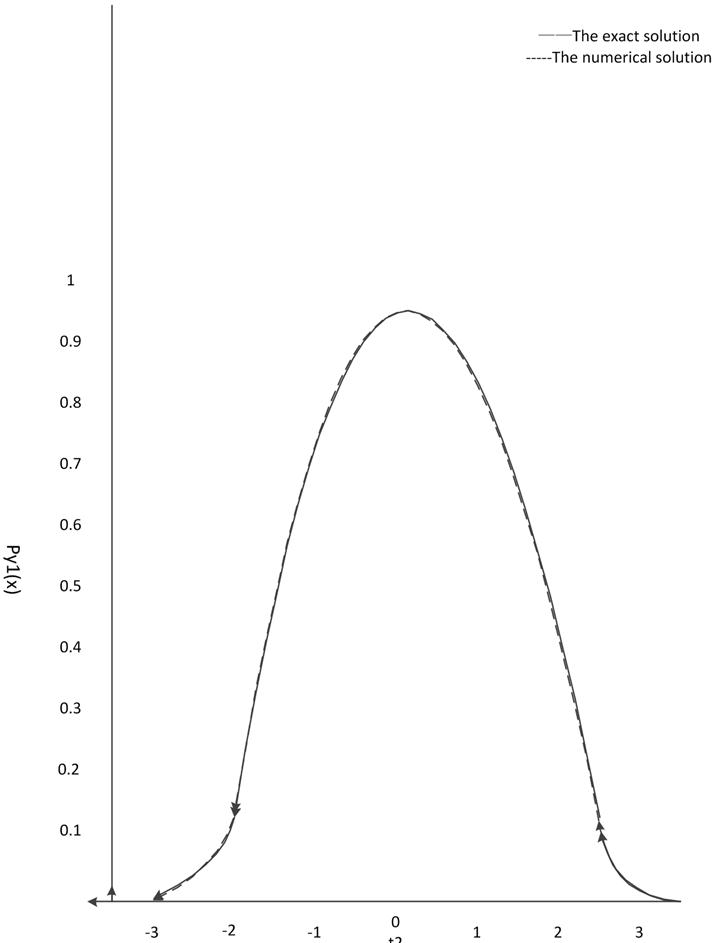Fig. 2(a). The exact solution and the numerical solution of displacement.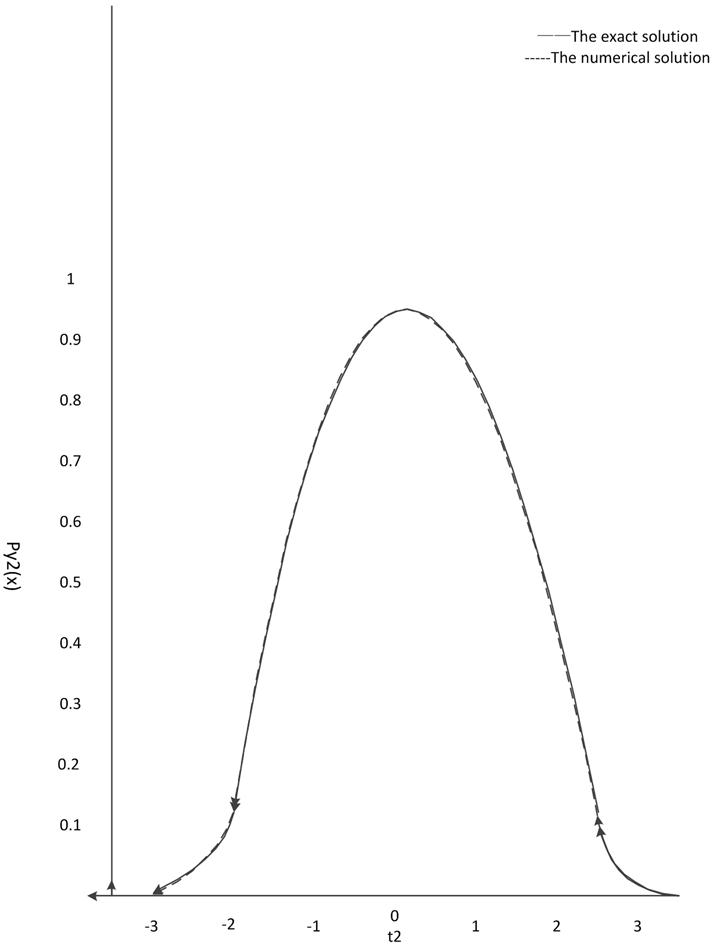Fig. 2(b). The exact solution and the numerical solution of velocity.

5. Conclusion

In this paper, the TVD Runge Kutta WENO difference scheme is obtained combing the third order TVD Runge Kutta method with the fifth order WENO scheme combined, is successfully applied to nonlinear dynamic system under the action of random excitation of, and the finite difference numerical solution of the FPK equation is obtained. It shows the validity and feasibility of the method, and overcomes the disadvantage of the common finite difference method. It can accurately obtain the smaller tail probability density and no oscillation, which is very important for the reliability analysis.

References

1. Y. K. Lin and G. Q. Cai. Probabilistic Structural Dynamics: Advanced Theory and Applications, 1995. (New York: MeGraw-Hill).
2. Zhu Weiqiu. Nonlinear Stochastic Dynamics and Controls---Frame of Hamilton Theory, 2003. (Beijing: Science Press) (in Chinese).
3. Zhao Chaoying, Tan Weihan and Guo Qizhi. The solution of the Fokker-Planck equation of non-degenerate parametric amplification system for generation of squeezed light, 2003 Acta Phys. Sin. 52 2694 (in Chinese).
4. Wang Ping, Yang Xine and Song X iaohui. Exact solution for a harmonic oscillator with a time-dependent inverse square potential by path-integral, 2003 Acta Phys. Sin. 52 2957 (in Chinese).
5. Xu Wei, He Qiu, Rong Haiwu and Fang Tong. Global analysis of stochastic bifurcation in a Duffing-van der Pol system, 2003 Acta Phys. Sin. 52 1365 (in Chinese).
6. Sun Zhongkui, Xu Wei and Yang Xiaoli. A new analytic approximate technique for strongly nonlinear dynamic systems. Journal of Dynamics and Control, 2005, 2 (3): 29-35 (in Chinese).
7. Liu Ruxun, Shu Qiwang. Some new methods in computational fluid dynamics [M]. Beijing: Science Press, 2003: 42-106.
8. Zhang Senwen. The solution of nonlinear staionary FPK equation using wavelet method [J]. Journal of Jinan University, 2002, 1: 29-33.(in Chinese).
9. Li Likang, Yu Chonghua, Zhu Zhenghua. Numerical method for Partial Differential Equation [M]. Shanghai: Fudan University Press, 1999. (in Chiinese).
10. Ye Dayi, Li Qingyang. Numerical method [M]. Beijing: High Education Press. (in Chinese).

 Contents 1. 2. 3. 3.1. 3.2. 3.3. 4. 5.
Article ToolsAbstractPDF(658K)